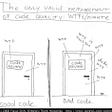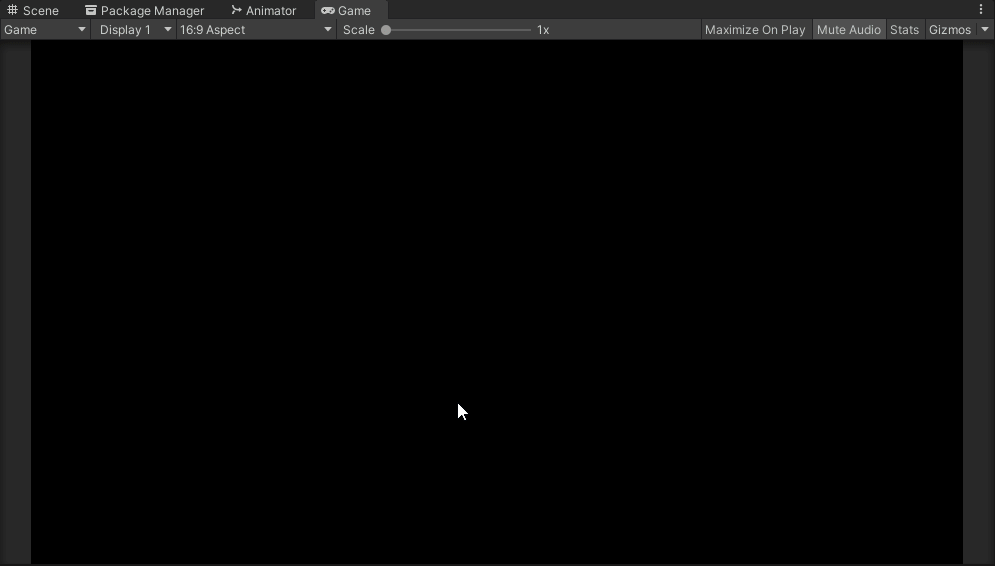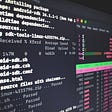# Number of Steps to Reduce a Number to Zero — Day 74(Python)

Today’s question is easy. I have taken this question from Leetcode’s daily coding challenge February edition.

1342. Number of Steps to Reduce a Number to Zero

Given a non-negative integer `num`, return the number of steps to reduce it to zero. If the current number is even, you have to divide it by 2, otherwise, you have to subtract 1 from it.

Example 1:

`Input: num = 14Output: 6Explanation: Step 1) 14 is even; divide by 2 and obtain 7. Step 2) 7 is odd; subtract 1 and obtain 6.Step 3) 6 is even; divide by 2 and obtain 3. Step 4) 3 is odd; subtract 1 and obtain 2. Step 5) 2 is even; divide by 2 and obtain 1. Step 6) 1 is odd; subtract 1 and obtain 0.`

Example 2:

`Input: num = 8Output: 4Explanation: Step 1) 8 is even; divide by 2 and obtain 4. Step 2) 4 is even; divide by 2 and obtain 2. Step 3) 2 is even; divide by 2 and obtain 1. Step 4) 1 is odd; subtract 1 and obtain 0.`

Example 3:

`Input: num = 123Output: 12`

Constraints:

• `0 <= num <= 10^6`

One of the ways to solve this problem is by checking how many times we have to divide the given number by 2. If we observe the example, we can see that whenever the division results in an odd number, the counter is incremented by 2, while for an even number the counter is incremented by 1. How can we check if the number is odd or even? Each time we divide the number by 2, we need to check the remainder. If we have a remainder, the number is odd else the number is even.

Let us look into the code snippet.

`class StepsCounter:    def numberOfSteps (self, num: int) -> int:        counter = 0        while(num > 1):            if num%2 == 0:                  counter += 1            else:                counter += 2            num = num//2        if num == 1:            counter += 1        return counter`

Complexity analysis.

Time Complexity

Since each time we are dividing by 2, the time complexity is O(logN).

Space Complexity.

Since we are not using any extra data structure to store intermediate results, the space complexity is O(1).

--

--

--

## More from Nerd For Tech

NFT is an Educational Media House. Our mission is to bring the invaluable knowledge and experiences of experts from all over the world to the novice. To know more about us, visit https://www.nerdfortech.org/.

## Kafka, Brod, and the Ig Nobel in C++ literature## InfluxDB Raspberry Pi Monitoring Cluster## Composing A Scene in Unity for Cutscenes## Lawsuits and Trials## Odoo 14 Installation On Ubuntu 20.04## What a beginner should know to become a Linux System Administrator## Annamariya Tharayil

Software Engineer. Find me @ www.linkedin.com/in/annamariya-jt

## Python Algorithms Every Programmer Should Know — Part 1## Guess my Number |Python Game## Glob Module in Python-Explained